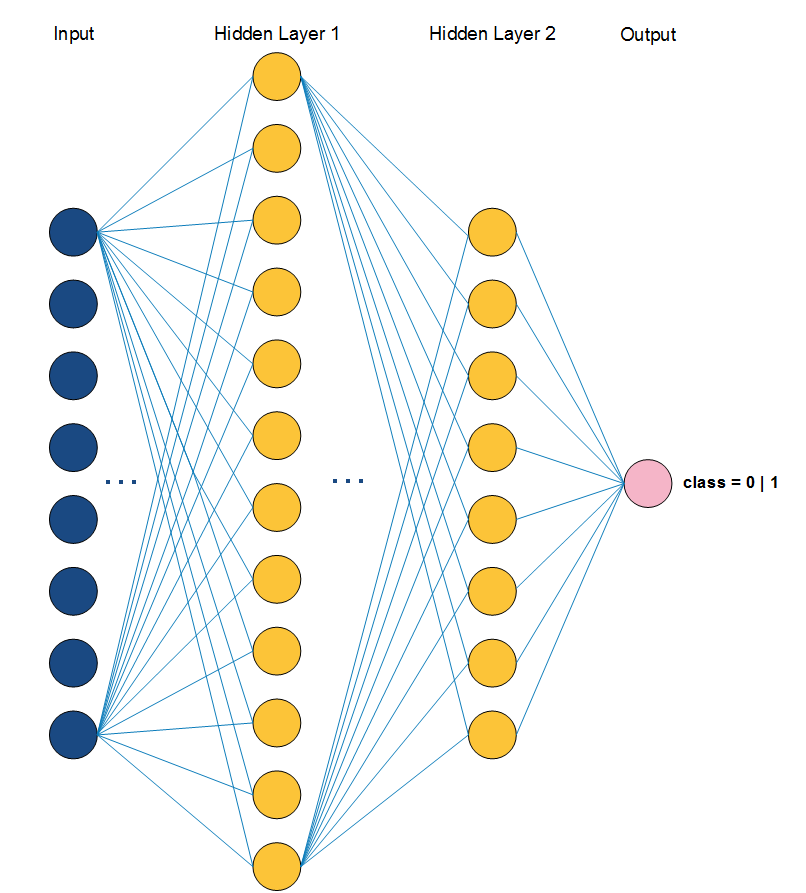## Introduction

There are many framework in working with Artificial Neural Networks (ANNs), for example, Torch, TensorFlow. In this tutorial, we present a framework, Theano, to create and evaluate ANN models in Microsoft Windows Environment.

Content

## Prerequisites

In order to work with this example program, and also to know how Theano works, you need to have the following prerequisites:

• Microsoft Windows 10 (Build 1607), aka Windows 10 Anniversary Update or higher versions
• Bash on Ubuntu on Windows must be enabled [Readme]
• Keras with Theano backend in virtual Ubuntu on Windows must be installed [Readme]
• Basic understanding of Python programming language [Readme]
• Basic understanding of machine learning, artificial neural network [ML | ANN]

## Your First Neural Network in Keras

In the following Python program, you will go through the steps to build and evaluate an ANN model on the `pima-indians-diabetes` dataset. The program includes 5 main steps as follows:

• Defining model
• Compiling model
• Inputing dataset into model
• Evaluating model
```              ```
# create first network with Keras
from keras.models import Sequential
from keras.layers import Dense
import numpy

# fix random seed for reproducibility
seed = 7
numpy.random.seed(seed)

# split into input (X) and output (Y) variables
X = dataset[:,0:8]
Y = dataset[:,8]

# create model
model = Sequential()

# compile model

# input the dataset into created model
model.fit(X, Y, nb_epoch=150, batch_size=10)

# evaluate the model
scores = model.evaluate(X, Y)
print("%s: %.2f%%" % (model.metrics_names, scores*100))
```
```The above image illustrates the defined `ANN` model. After programming your prefer `ANN` model, now, compile and run it in Keras environment by using the following steps:
• Open `Bash on Ubuntu on Windows` and change the directory to the project location. You need to put both the program and dataset in the same location.
• Type the command `workon keras`
• Then, `python sample.py`

## Handwritten Digit Recognition

At this point, you completed your first beginning program with Keras. Now, let's dive into something interested, computer vision. We present a basic demo with Convolutional Neural Network (CNN) with handwritten digit recognition problem. The program is available at this repository, named `mnist_cnn.py`.
There are few standard datasets in digit recognition problem, thus, in this tutorial, we use the MNIST dataset, which contains 70,000 images of handwritten numbers from 0 to 9. The digit in each image has been size-normalized and centered in a fixed-size.
Like your first program, in this example, first, we need to read the input dataset. Each image is represented as matrix with `28 x 28` dimension. Next, we define size of pooling area for max pooling. Then, define the number of convolutional filters (feature detectors) to be used and the size of them also.

```            ```
# input image dimensions
img_rows, img_cols = 28, 28
# number of convolutional filters to use
nb_filters = 32
# convolution kernel size
kernel_size = (3, 3)
# size of pooling area for max pooling
pool_size = (2, 2)
```
```
Second, we reshape all image to `28 x 28` dimension by calling the defined `reshape` function in Keras (in line 35). The function contains four arguments `(samples, channels, height, width)`, where `channels` is `0` or `3`, which means, gray-scale or RGB mode, respectively.
Third, we define the CNN model as follows:
```            ```
# define model
model = Sequential()
border_mode='valid',
input_shape=input_shape))

# compile model
model.compile(loss='categorical_crossentropy',
metrics=['accuracy'])
```
```
Forth, after define the model, now input the dataset into it and then, run and evaluate. The `nb_epoch` affects the time-consuming when running this example, we recommend to edit `nb_epoch` to a lower number when running time is too long.
```            ```
# input the dataset
model.fit(X_train, Y_train,
batch_size=batch_size,
nb_epoch=nb_epoch,
verbose=1,
validation_data=(X_test, Y_test))
# evaluate model
score = model.evaluate(X_test, Y_test, verbose=0)
print('Test score:', score)
print('Test accuracy:', score)
```
```